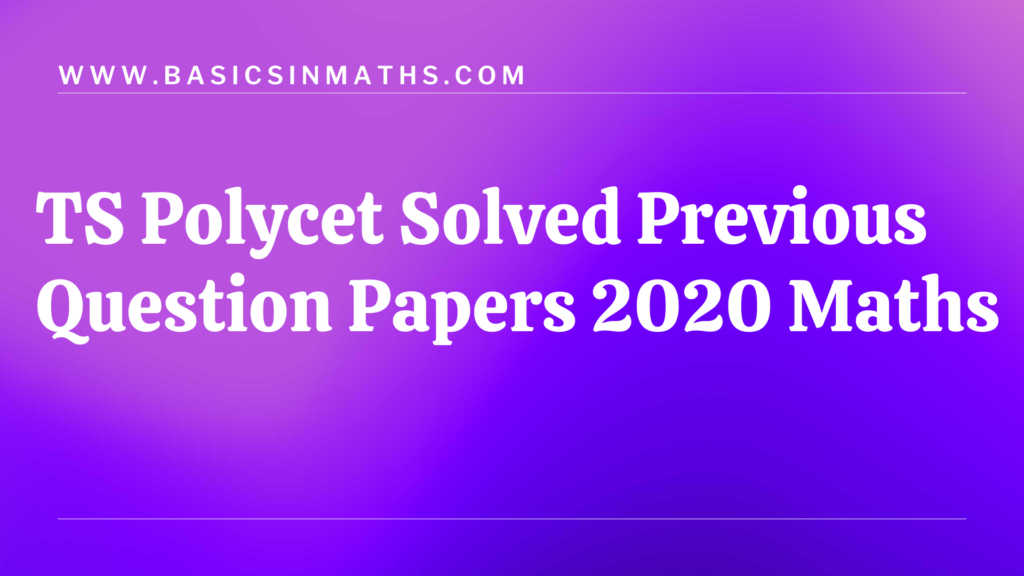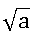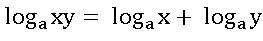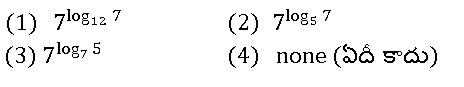Welcome To Basics In Maths

# Maths TS Polycet || Solved Previous Question Papers 2020# TS Polycet || Solved Previous Question Papers 2020 Math

## The syllabus of  Maths, Physics, Chemistry and Biology for POLYCET-2022 is the same as that of SSC Examination conducted by the Board of Secondary Education, Telangana.

##### TS Polycet || Solved Previous Question Papers 2021 Mathematics gives an idea becuase,  it is very helpful to solve the problems in POLYCET entence  examination

TS VI – IX Maths Concepts

#### Chapter 1: Real Numbers

1.If 7 divides a2 then

a2  ను 7 భాగించినచో

(1)  7 divides a (a ను 7 భాగిస్తుంది)

(2) 7 divides(ను 7 భాగిస్తుంది)

(3) a divide 7 (7 ను a భాగిస్తుంది)

(4) none (ఏదీ కాదు)

2. In the formula, which of the following is true?అయిన, ఈ క్రింది వాటిలో ఏది సత్యము.

(1)  x > 0, y > 0, a = 1

(2) x < 0, y < 0, a = 1

(3)  a > 0, y > 0, x = 1

(4) x > 0, y > 0, a ≠ 1

` `

#####` `

##### (3)  5                  (4) 2

` `

###### (3) c2       (4) None(ఏది కాదు)

` `